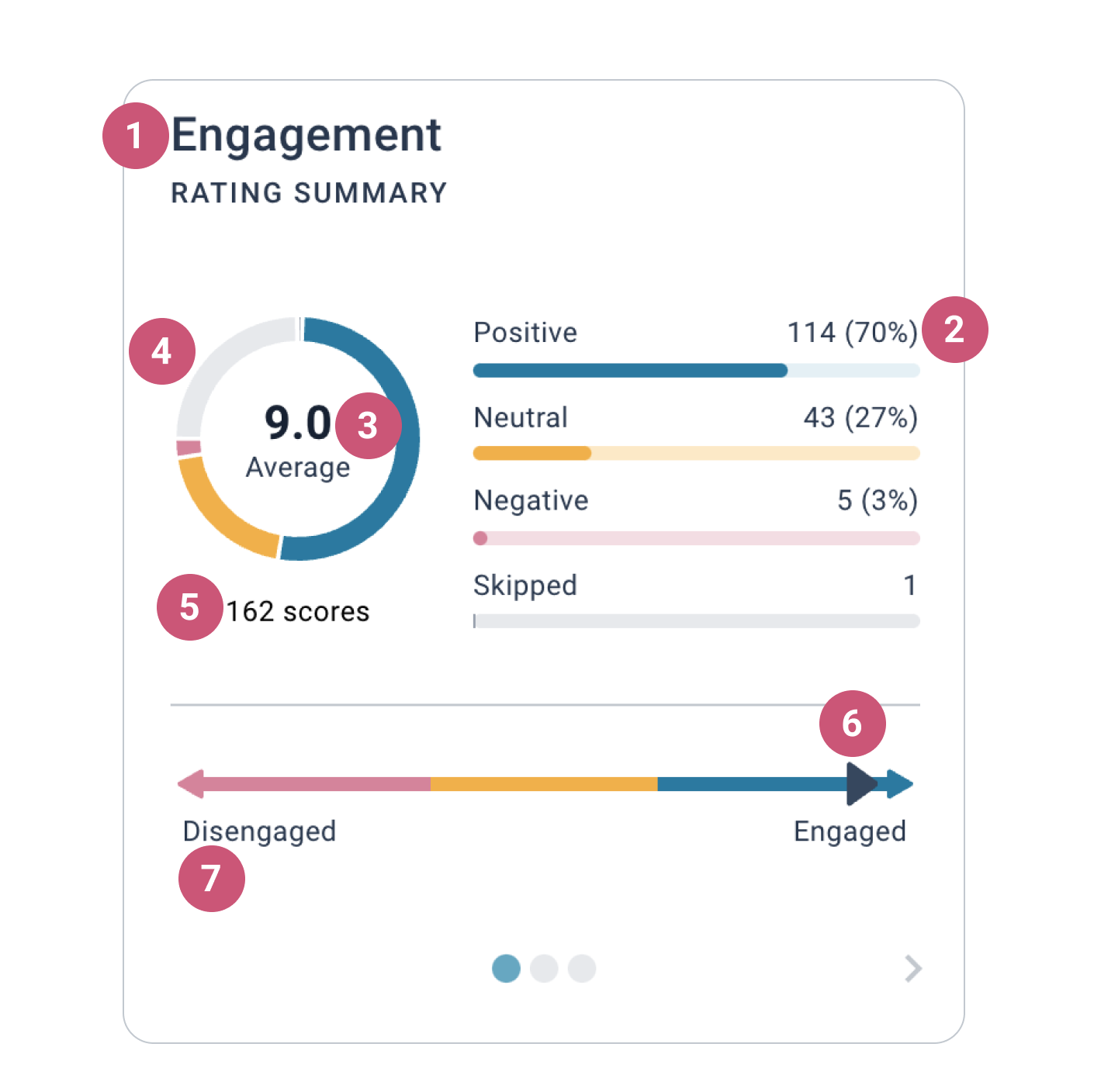Help Center
1. Help Center
2. How to use Joyous
3. Understanding Insights

# Understanding the rating summary

📺  Watch the video lesson here: Understanding insights

### What is shown on the summary

The rating summary consists of seven key pieces of information.1. The category name.

2. The distribution of scores.

• Positive. This is the number of 9-10 ratings as a % of all ratings for rated statements in the category.
• Neutral. This is the number of 7-8 ratings as a % of all ratings for rated statements in the category.
• Negative. This is the number of 0-6 ratings as a % of all ratings for rated statements in the category.
• Skipped. The number of questions where the skip question option was selected.

3. The category average score.

• We use a simple average to calculate scores.
• Questions and topics are not weighted.
• Each individual rating contributes equally to an average score. For example:  (7 + 4 + 10 + 0 + 2 + 5) / 6 = 4.7

📝  Note: If the same question has been asked more than once over time, only the most recent round's ratings will be included in the average score calculation.

4. Unanswered rated statements. The proportion of rated statements in the category that have not been answered (i.e. non-participation).

5. Answered rated statements. This is the total number of rated statements in the category that received an answer.

6. The trend over time.

• The category trend compares the scores of the latest two questions in the category.
• If the arrow is pointing to the right, the latest question in the category has a higher score than the previous question in the category.
• If the arrow is pointing to the left, the latest question in the category has a lower score than the previous question in the category.
• If you see a dot, there is no change between the scores of the current question and the score of the previous question in the category.

7. Spectrum labels.

• The left label (Disengaged) is the category spectrum low.
• The right label (Engaged) is the category spectrum high.